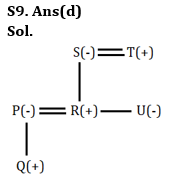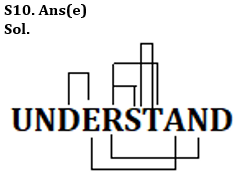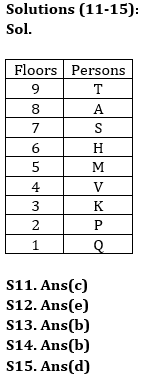Latest Banking jobs   »

# Reasoning Quiz For Bank of Baroda AO 2023-20th April

Directions (1-5): In these questions, relationship between different elements is shown in the statements. The statements are followed by conclusions.
(a) If only conclusion I is true
(b) If only conclusion II is true
(c) If either conclusion I or II is true
(d) If neither conclusion I nor II is true
(e) If both conclusions I and II are true

Q1. Statements: X≥U=S≥J≥O≥K<P
Conclusions I: X>O II: O=X

Q2. Statements: M>S≥O=P≤K<G>R<I
Conclusion I: P≤M II: O<G

Q3. Statements: C=S≤E≤ Y=X>D>F≥L
Conclusion I: X≥C II: L<Y

Q4. Statements: S>U>T>M=P≥R
Conclusion I: P<U II: M>R

Q5. Statements: Y≥M=Q<Z≤I =W
Conclusion I: W>M II: Y>Z

Q6. In an exam M, N, O, P and Q score different marks. M score less than the only O. P score less than N. The one who score lowest marks got twelve marks. P does not score marks that are an even number. If N’s marks are 20 then what will be the possible marks of P?
(a) 14
(b) 10
(c) 21
(d) 17
(e) 22

Q7. If in the number 2975831, 2 is subtracted from each of the digit which is greater than 6 and 1 is added to each of the digit which is less than 6 then how many digits are repeated in the number thus formed?
(a) One
(b) Two
(c) Three
(d) None
(e) Four

Q8. Which of the following would replace @ and & respectively in the following expression so that F ≤ Q, Q>G holds true?
Q ≥ T # M = D > G, N < T @ U ≥ F = E
(a) >, ≤
(b) ≥, >
(c) ≥, <
(d) >, =
(e) None of these

Q9. There are six members in a family. P is mother of Q. R is son of S. U is daughter of S. T is father of U. Q is son of R. How is Q related to T?
(a) Son
(b) Daughter
(c) Sister
(d) Grandson
(e) Can’t be determined

Q10. How many pairs of letters are there in the word UNDERSTAND, each of which have as many letters between (in both forward and backward direction) them in the word as they have between them in the English alphabetical series?
(a) Three
(b) Four
(c) More than Six
(d) Five
(e) Six

Directions (11-15): Study the information carefully and answer the questions given below.
Nine persons live at nine different floor of the building in such a way that ground floor is numbered as 1st, floor just above it is numbered as 2nd and so on till the topmost floor is numbered as 9th.
P lives at an even number floor. Not more than five persons live below P. Three persons live between P and H. V live just above K and just below M. K does not live just above H. Three persons live between M and T. S lives just below A. Q does not live just above and just below H. T does not live on the ground floor.

Q11. Who among the following persons lives at topmost floor?
(a) Q
(b) M
(c) T
(d) A
(e) None of these

Q12. Who among the following persons lives at 6th floor?
(a) V
(b) P
(c) S
(d) M
(e) H

Q13. Who among the following persons lives just below P?
(a) K
(b) Q
(c) M
(d) T
(e) None of these

Q14. How many persons live between S and P?
(a) None
(b) Four
(c) More than Four
(d) Two
(e) Three

Q15. Four of the following five are alike in certain way based from a group, find the one that does not belong to that group?
(a) A
(b) H
(c) V
(d) K
(e) P

Solutions

Solution (1-5):
S1. Ans. (c)
Sol. I: X>O(false) II: O=X(false)

S2. Ans. (b)
Sol. I: P≤M (false) II: O<G(true)

S3. Ans. (e)
Sol. I: X≥C (true) II: L<Y(false)

S4. Ans. (a)
Sol. I: P<U(true) II: M>R(false)

S5. Ans. (a)
Sol. I: W>M(true) II: Y>Z(false)

S6. Ans. (d)
Sol. O> M > N (20) > P > Q (12)

S7. Ans.(a)
Sol. Original number- 2975831
Obtained number- 3756642

S8. Ans(b)## FAQs

### What is the selection process of Bank of Baroda AO 2023?

The selection process of Bank of Baroda AO consists of Online exam and Interview

#### Congratulations!Union Budget 2023-24: Free PDF